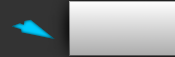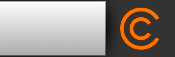Register

## Get Key Troubles

Discuss issues related to the Casio Basic Language
Member
Posts: 48
Joined: Mon Apr 23, 2012 3:58 am
Location: Chennai,India
Calculators: Casio fx-9750 G II, Casio fx-9860G Slim, Casio fx-9860 GII

### Get Key Troubles

Hi,

Am having a trouble with getkey.

Here's my code:
"KM"?->K
Do:Getkey
LpWhile Not Ans
If Ans>=25
Then Ans=76=>K+0.01->K
Ans=66=>K+0.02->K
Ans=56=>K+0.05->K
Ans=46=>K+0.1->K
Ans=36=>K+0.2->K
Ans=26=>K+0.5->K
IfEnd.

I need this fuction to work while pressing the keys.
If I press the numbers it should ask for the "KM" value and then should display the result.
Pls help.Posts: 89
Joined: Thu Apr 05, 2012 3:16 pm
Location: Akron, OH, USA
Calculators: Casio fx-9750 G II, Casio fx-CG 10
nagarajan wrote:Hi,

Am having a trouble with getkey.

Here's my code:
"KM"?->K
Do:Getkey
LpWhile Not Ans
If Ans>=25
Then Ans=76=>K+0.01->K
Ans=66=>K+0.02->K
Ans=56=>K+0.05->K
Ans=46=>K+0.1->K
Ans=36=>K+0.2->K
Ans=26=>K+0.5->K
IfEnd.

I need this fuction to work while pressing the keys.
If I press the numbers it should ask for the "KM" value and then should display the result.
Pls help.

This is better, i think... but I don't think I really understand what you want to do

Code: Select all
`DoLpWhile not GetkeyIf Ans>=25 Then Ans=76=>K+0.01->K Ans=66=>K+0.02->K Ans=56=>K+0.05->K Ans=46=>K+0.1->K Ans=36=>K+0.2->K Ans=26=>K+0.5->K IfEnd."KM" -> K`
http://casio.clrhome.org
http://clrhome.org/http://tny.im/ikMember
Posts: 48
Joined: Mon Apr 23, 2012 3:58 am
Location: Chennai,India
Calculators: Casio fx-9750 G II, Casio fx-9860G Slim, Casio fx-9860 GII
flyingfisch wrote:This is better, i think... but I don't think I really understand what you want to do

Code: Select all
`DoLpWhile not GetkeyIf Ans>=25 Then Ans=76=>K+0.01->K Ans=66=>K+0.02->K Ans=56=>K+0.05->K Ans=46=>K+0.1->K Ans=36=>K+0.2->K Ans=26=>K+0.5->K IfEnd."KM" -> K`

Thanks for the idea.
What i need is:
1.Inputting K value.
2.After the input it should keep on adding .01,.02,.05,.1,.2 etc while pressing the keys assigned to the function.

Senior MemberPosts: 66
Joined: Sun Apr 15, 2012 4:00 pm
Calculators: Casio fx-9860G
lbl 1
do
getkey
lpwhile ans=0
Ans=66=>blabla
Goto 1

Or you can make an infinite loop
do
getkey
ans=66=> blabla
ans=47=> Goto Q
lpwhile 1
lbl Q
Locate 1,1,"EXIT"

MemberPosts: 27
Joined: Mon Apr 09, 2012 7:09 pm
Location: France
nagarajan wrote:Hi,

Am having a trouble with getkey.

Here's my code:
"KM"?->K
Do:Getkey
LpWhile Not Ans
If Ans>=25
Then Ans=76=>K+0.01->K
Ans=66=>K+0.02->K
Ans=56=>K+0.05->K
Ans=46=>K+0.1->K
Ans=36=>K+0.2->K
Ans=26=>K+0.5->K
IfEnd.

I need this fuction to work while pressing the keys.
If I press the numbers it should ask for the "KM" value and then should display the result.
Pls help.

The user input "MK" value and press EXE to validate. So the GetKey juste after return obviously 31 (EXE).
You must wait until the button is released. (While Getkey:WhileEnd)

Member
Posts: 48
Joined: Mon Apr 23, 2012 3:58 am
Location: Chennai,India
Calculators: Casio fx-9750 G II, Casio fx-9860G Slim, Casio fx-9860 GII
PierrotLL wrote:The user input "MK" value and press EXE to validate. So the GetKey juste after return obviously 31 (EXE).
You must wait until the button is released. (While Getkey:WhileEnd)

Can you explain this with the sample code pls?

Senior MemberPosts: 66
Joined: Sun Apr 15, 2012 4:00 pm
Calculators: Casio fx-9860G
Code: Select all
`"KM"?->KLbl 1DoFor 1->A To 75:NextGetkeyLpWhile Ans=0ClrtextLocate 1,1,KAns=79=>K+0,02->KAns=47=>Goto QGoto 1Lbl QClrTextLocate 4,5,"Quit"`

Play around with this working program.

MemberPosts: 27
Joined: Mon Apr 09, 2012 7:09 pm
Location: France
Code: Select all
`"KM"?->KWhile GetKey:WhileEnd [color="#008000"]//wait while a key is pressed[/color]Do:GetkeyLpWhile Not AnsAns=76=>K+0.01->K //your If statement was uselessAns=66=>K+0.02->KAns=56=>K+0.05->KAns=46=>K+0.1->KAns=36=>K+0.2->KAns=26=>K+0.5->K`

@aapman55 : your code will slow-down the program on old calcs, and the result will be wrong if the user press EXE a long time. A simple While is better.

Senior MemberPosts: 66
Joined: Sun Apr 15, 2012 4:00 pm
Calculators: Casio fx-9860G
thanks pierrot never thought of that wait till key is pressed part, because i thought it wouldnt work.